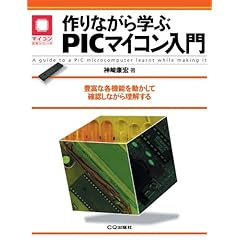## 記事一覧

### シフトレジスタ(74HC164)その３

2009.07.21

データシートのタイミングチャートを見ると、このICの面白い特性が分かります。CLRとBピンがH状態の時、クロックと同期を取ったAピンの立ち上がりでQAがHとなり、以後クロックの上げ下げに応じてH状態がQA=>QB=>QCとシフトしていきます。
この機能を応用して、例えば"00001101"の状態を作り出すには、タイミングチャートのように、Aピンを2クロック分H、1クロックL、1クロックHとし、あと4クロック分Lを送れば実現されます。
クロックの変化がない限り状態は保持され、またクロックの周期はランダム(1usとか10ms)でもOKです。

これだけの操作を、PICからはたった2本のI/Oピンで制御できるのです。

### UBW基板

2009.07.17PIC18F2550で作成したUBW基板です。
ベースとなるPICのプログラム「ファームウエア」を書き込むことで、後はUSB経由でのプログラミングが可能となります。
UBWに関しては千秋ゼミさんの解説がかなり具体的で詳細です。### 作りながら学ぶPICマイコン入門

2009.07.16CCP、USART、A/DなどPICの機能について幅広く解説されているので、ミッドレンジPICの使いこなしには欠かせない一冊です。

タグ：PIC 勉強 参考書

### シフトレジスタ(74HC164)その２

2009.07.15

シフトレジスタで遊んでみました。

`program SHIFREGdim i, j, k as byteconst pp=50main:    TRISIO = %00000000    'OPTION_REG.NOT_GPPU = 0    WPU        = %00000100    GPIO     = %00000000    do        'CLEAR        GPIO.4 = 0        for i = 0 to 8                GPIO.2 = 1                GPIO.2 = 0                delay_ms(pp)        next i        delay_ms(pp*2)                'LEFT > RIGHT        GPIO.4 = 1        for i = 0 to 8                GPIO.2 = 1                GPIO.4 = 0                GPIO.2 = 0                delay_ms(pp)        next i        delay_ms(pp*2)                'RIGHT > LEFT        for j = 0 to 8            GPIO.4 = 1            for i = j to 8                    GPIO.2 = 1                    GPIO.4 = 0                    GPIO.2 = 0            next i            delay_ms(pp)            for i= 8-j to 8                    GPIO.2 = 1                    GPIO.2 = 0            next i        next j        delay_ms(pp*2)        delay_ms(pp*2)        'LEFT > RIGHT FILL        GPIO.4 = 1        for i = 0 to 7                GPIO.2 = 1                GPIO.2 = 0                delay_ms(pp)        next i        delay_ms(pp*2)        'ERASE        GPIO.4 = 0        for i = 0 to 7                GPIO.2 = 1                GPIO.2 = 0                delay_ms(pp)        next i        delay_ms(pp*2)        'LEFT > RIGHT FILL        for k = 0 to 8                for j = 0 to k                    GPIO.4 = 1                    GPIO.2 = 1                    GPIO.2 = 0                next j                GPIO.4 = 0                for i = j to 7                     GPIO.2 = 1                     GPIO.2 = 0                next i             delay_ms(pp)         next k         delay_ms(pp*2)        'LEFT > RIGHT ERASE        for k = 0 to 7                for j = 0 to 7 - k                    GPIO.4 = 1                    GPIO.2 = 1                    GPIO.2 = 0                next j             delay_ms(pp)                GPIO.4 = 0                for i = 0 to 7                     GPIO.2 = 1                     GPIO.2 = 0                next i         next k        delay_ms(pp*2)        'FLASH        for k = 0 to 7                for j = 0 to 7                    GPIO.4 = 1                    GPIO.2 = 1                    GPIO.2 = 0                next j                delay_ms(pp*2)                for i = 0 to 7                     GPIO.4 = 0                     GPIO.2 = 1                     GPIO.2 = 0                next i                delay_ms(pp*2)         next k        delay_ms(pp*2)    loop until 0 = 1end.`

Mikrobasicにてプログラミング。この手の実験的な軽い開発には便利なツールですね。

シフトレジスタは左から右への一方向へのシフトしかできないので、反対方向に流すにはコツがいります。

### ドットマトリックスLED時計の製作④

2009.07.13スモークがかってるのでLEDのコントラストも上がり見やすいです。